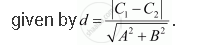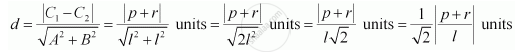CBSE (Arts) Class 11CBSE
Share

# Find the Distance Between Parallel Lines L (X + Y) + P = 0 And L (X + Y) – R = 0 - CBSE (Arts) Class 11 - Mathematics

ConceptDistance of a Point from a Line

#### Question

Find the distance between parallel lines  (y) + = 0 and (y) – = 0

#### Solution

It is known that the distance (d) between parallel lines ABC1 = 0 and ABC2 = 0 isThe given parallel lines are (y) + = 0 and (y) – = 0.

lx ly + = 0 and lx ly – r = 0

Here, A = lB = lC1 = p, and C= –r.

Therefore, the distance between the parallel lines isIs there an error in this question or solution?

#### Video TutorialsVIEW ALL 

Solution Find the Distance Between Parallel Lines L (X + Y) + P = 0 And L (X + Y) – R = 0 Concept: Distance of a Point from a Line.
S